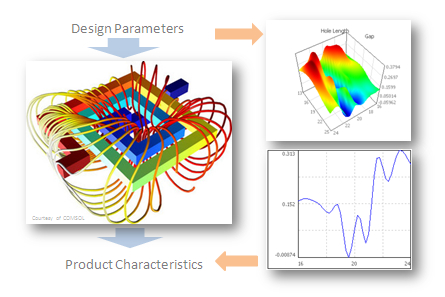﻿ Meta-Modeling

CAD/CAE Design Technology for Reliability and Quality

Meta-Modeling

There are 2 different ways to create the model codes, its advantages and disadvantages of which complement each other. The combination of both methods brings advances and undreamt-of possibilities for modeling real objects. OptiY Software offers this approach and it is therefore an indispensable tool for simulation engineers.

 Physics Model Meta Model Mathematical formulas to describe physical laws in the form of differential and integral equations. Numerical algorithms for solving such systems of equations. Long development time of individual equations from the real object to the finished model codes. Efficient systems of equations to describe the real object behavior, but usually only serial implementation of the algorithms with long computing time. Verification between model and real object is only possible through little-targeted measurement data. Code-export Only measurement data from the real object or test pattern. Physical working principles and laws are completely unknown and not required. Machine learning (clustering, regression and classification) for the automatic recognition of mathematical relationships between input and output parameters. Extremely fast development time and semi-automatic generation of model codes. Completely parallel implementation of algorithms for real-time computing. Verification between model and real object is only possible through a large amount of measurement data. Code-export in C/C++, Matlab, Modelica, VB

Physical model is used as object for data generation. Numerous commercial simulation systems can be coupled with OptiY. Using of statistical design of experiments, the entire parameter space is stretched and calculated. With these data obtained, accurate metamodel or surrogate model can be mapped using machine learning.

If the data does not have the required quantity to generate accurate metamodels, additional data points can be generated with the help of coupled physics model. Using of adaptive design of experiments from OptiY, which are based on existing data, new targeted data points can be very efficiently proposed and calculated by the physics model. Measurement data can then be mapped together with newly additional simulation data in sufficient quantities to accurate metamodel.